Mechanical Engineering - ME 2015 GATE Paper (Practice Test)

# Mechanical Engineering - ME 2015 GATE Paper (Practice Test)

Test Description

## 65 Questions MCQ Test GATE Mechanical (ME) 2023 Mock Test Series | Mechanical Engineering - ME 2015 GATE Paper (Practice Test)

Mechanical Engineering - ME 2015 GATE Paper (Practice Test) for Mechanical Engineering 2023 is part of GATE Mechanical (ME) 2023 Mock Test Series preparation. The Mechanical Engineering - ME 2015 GATE Paper (Practice Test) questions and answers have been prepared according to the Mechanical Engineering exam syllabus.The Mechanical Engineering - ME 2015 GATE Paper (Practice Test) MCQs are made for Mechanical Engineering 2023 Exam. Find important definitions, questions, notes, meanings, examples, exercises, MCQs and online tests for Mechanical Engineering - ME 2015 GATE Paper (Practice Test) below.
Solutions of Mechanical Engineering - ME 2015 GATE Paper (Practice Test) questions in English are available as part of our GATE Mechanical (ME) 2023 Mock Test Series for Mechanical Engineering & Mechanical Engineering - ME 2015 GATE Paper (Practice Test) solutions in Hindi for GATE Mechanical (ME) 2023 Mock Test Series course. Download more important topics, notes, lectures and mock test series for Mechanical Engineering Exam by signing up for free. Attempt Mechanical Engineering - ME 2015 GATE Paper (Practice Test) | 65 questions in 180 minutes | Mock test for Mechanical Engineering preparation | Free important questions MCQ to study GATE Mechanical (ME) 2023 Mock Test Series for Mechanical Engineering Exam | Download free PDF with solutions
 1 Crore+ students have signed up on EduRev. Have you?
Mechanical Engineering - ME 2015 GATE Paper (Practice Test) - Question 1

### Q. No. 1 – 5 Carry One Mark Each What is the adverb for the given word below? Misogynous

Mechanical Engineering - ME 2015 GATE Paper (Practice Test) - Question 2

### Ram and Ramesh appeared in an interview for two vacancies in the same department. The probability of Ram?s selection is 1/6 and that of Ramesh is 1/8. What is the probability that only one of them will be selected?

Detailed Solution for Mechanical Engineering - ME 2015 GATE Paper (Practice Test) - Question 2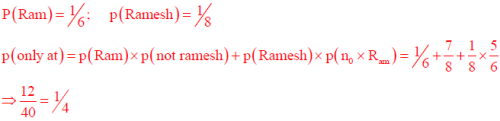Mechanical Engineering - ME 2015 GATE Paper (Practice Test) - Question 3

### Choose the appropriate word/phrase, out of the four options given below, to complete the following sentence: Dhoni, as well as the other team members of Indian team, _____ present on the occasion.

Mechanical Engineering - ME 2015 GATE Paper (Practice Test) - Question 4

An electric bus has onboard instruments that report the total electricity consumed since the start of the trip as well as the total distance covered. During a single day of operation, the bus travels on stretches M, N, O and P, in that order. The cumulative distances travelled and the corresponding electricity consumption are shown in the table below.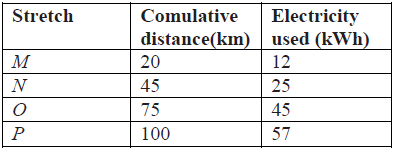The stretch where the electricity consumption per km is minimum is

Detailed Solution for Mechanical Engineering - ME 2015 GATE Paper (Practice Test) - Question 4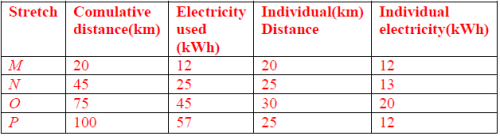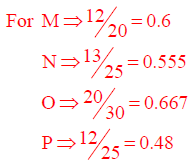Mechanical Engineering - ME 2015 GATE Paper (Practice Test) - Question 5

Choose the word most similar in meaning to the given word:

Awkward

Mechanical Engineering - ME 2015 GATE Paper (Practice Test) - Question 6

Q. No. 6 – 10 Carry Two Marks Each

In the following sentence certain parts are underlined and marked P, Q and R. One of the parts may contain certain error or may not be acceptable in standard written communication.

Select the part containing an error. Choose D as your Answer: if there is no error.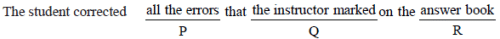Detailed Solution for Mechanical Engineering - ME 2015 GATE Paper (Practice Test) - Question 6

The is not required in „Q?

Mechanical Engineering - ME 2015 GATE Paper (Practice Test) - Question 7

Given below are two statements followed by two conclusions. Assuming these statements to be true, decide which one logically follows.

Statement:

I. All film stars are playback singers.

II. All film directors are film stars.

Conclusions:

I. All film directors are playback singers.

II. Some film stars are film directors.

Mechanical Engineering - ME 2015 GATE Paper (Practice Test) - Question 8

Lamenting the gradual sidelining of the arts in school curricula, a group of prominent artists wrote to the Chief Minister last year, asking him to allocate more funds to support arts education in schools. However, no such increase has been announced in this year?s Budget. The artists expressed their deep anguish at their request not being approved, but many of them remain optimistic about funding in the future.

Which of the statement(s) below is/are logically valid and can be inferred from the above statements?

i. The artists expected funding for the arts to increase this year.

ii. The Chief Minister was receptive to the idea of increasing funding for the arts.

iii. The Chief Minister is a prominent artist.

iv. Schools are giving less importance to arts education nowadays.

Mechanical Engineering - ME 2015 GATE Paper (Practice Test) - Question 9

If a2 + b2 + c2 +1, then ab + bc + ac lies in the interval

Detailed Solution for Mechanical Engineering - ME 2015 GATE Paper (Practice Test) - Question 9

Given that
a^2 + b^2 + c^2 = 1

Therefore,
1 + 2(ab + bc + ca) ≥ 0_________ { by (a+b+c)^2 = a^2 + b^2 + c^2 + 2(ab + bc + ca)}

ab + bc + ca ≥ -1/2

(a-b)^2 + (b-c)^2 + (c-a)^2 ≥ 0
2 [ a^2 + b^2 + c^2 - ab - bc - ca ] ≥ 0
2 [ 1 - (ab + bc + ca)] ≥ 0
Therefore, 1 ≥ (ab + bc + ca)

so, ur answer is [-1/2, 1]

*Answer can only contain numeric values
Mechanical Engineering - ME 2015 GATE Paper (Practice Test) - Question 10

A tiger is 50 leaps of its own behind a deer. The tiger takes 5 leaps per minute to the deer?s 4. If the tiger and the deer cover 8 metre and 5 metre per leap respectively, what distance in meters will the tiger have a run before it catches the deer?

Detailed Solution for Mechanical Engineering - ME 2015 GATE Paper (Practice Test) - Question 10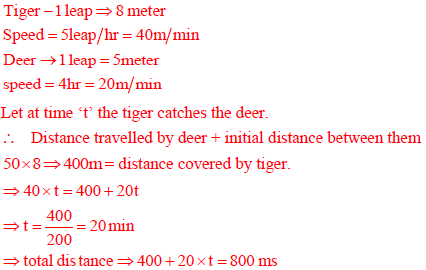Mechanical Engineering - ME 2015 GATE Paper (Practice Test) - Question 11

Q. No. 11 – 35 Carry One Mark Each

If any two columns of a determinant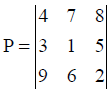are interchanged, which one of the following statements regarding the value of the determinant is CORRECT?

*Answer can only contain numeric values
Mechanical Engineering - ME 2015 GATE Paper (Practice Test) - Question 12

A wheel of radius r rolls without slipping on a horizontal surface shown below. If the velocity of point P is 10 m/s in the horizontal direction, the magnitude of velocity of point Q (in m/s) is ______.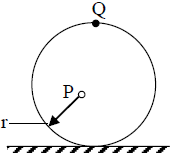(Important - Enter only the numerical value in the answer)

Detailed Solution for Mechanical Engineering - ME 2015 GATE Paper (Practice Test) - Question 12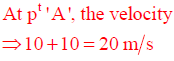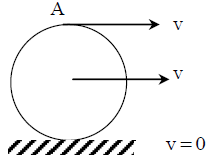Mechanical Engineering - ME 2015 GATE Paper (Practice Test) - Question 13

Which one of the following types of stress-strain relationship best describes the behavior of brittle materials, such as ceramics and thermosetting plastics, (σ = stress and ε = strain)?

Mechanical Engineering - ME 2015 GATE Paper (Practice Test) - Question 14

The function of interpolator in a CNC machine controller is to

Mechanical Engineering - ME 2015 GATE Paper (Practice Test) - Question 15

Holes of diameter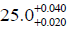mm are assembled interchangeably with the pins of diameter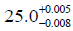mm.

The minimum clearance in the assembly will be

Detailed Solution for Mechanical Engineering - ME 2015 GATE Paper (Practice Test) - Question 15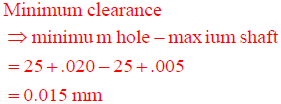Mechanical Engineering - ME 2015 GATE Paper (Practice Test) - Question 16

Simpson?s 1/3 rule is used to integrate the function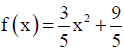between x = 0 and x = 1 using the least number of equal sub-intervals. The value of the integral is ___________

(Important - Enter only the numerical value in the answer)

Detailed Solution for Mechanical Engineering - ME 2015 GATE Paper (Practice Test) - Question 16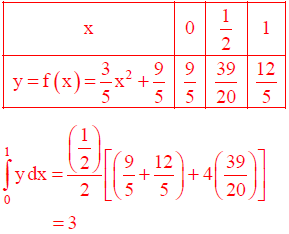Mechanical Engineering - ME 2015 GATE Paper (Practice Test) - Question 17

Consider fully developed flow in a circular pipe with negligible entrance length effects. Assuming the mass flow rate, density and friction factor to be constant, if the length of the pipe is doubled and the diameter is halved, the head loss due to friction will increase by a factor of

Detailed Solution for Mechanical Engineering - ME 2015 GATE Paper (Practice Test) - Question 17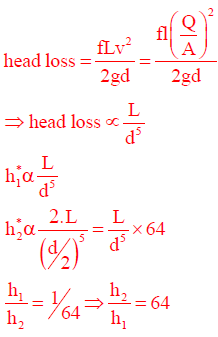Mechanical Engineering - ME 2015 GATE Paper (Practice Test) - Question 18

For an ideal gas with constant values of specific heats, for calculation of the specific enthalpy,

*Answer can only contain numeric values
Mechanical Engineering - ME 2015 GATE Paper (Practice Test) - Question 19

A Carnot engine (CE-1) works between two temperature reservoirs A and B, where TA = 900 K and TB = 500 K. A second Carnot engine (CE-2) works between temperature reservoirs B and C, where TC = 300 K. In each cycle of CE-1 and CE-2, all the heat rejected by CE-1 to reservoir B is used by CE-2. For one cycle of operation, if the net Q absorbed by CE-1 from reservoir A is 150 MJ, the net heat rejected to reservoir C by CE-2 (in MJ) is _________.

(Important - Enter only the numerical value in the answer)

Detailed Solution for Mechanical Engineering - ME 2015 GATE Paper (Practice Test) - Question 19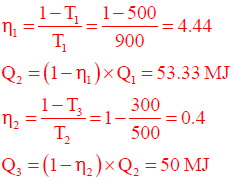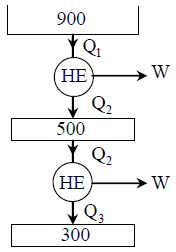*Answer can only contain numeric values
Mechanical Engineering - ME 2015 GATE Paper (Practice Test) - Question 20

A stream of moist air (mass flow rate = 10.1 kg/s) with humidity ratio of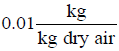mixes with a second stream of superheated water vapour flowing at 0.1 kg/s. Assuming proper and uniform mixing with no condensation, the humidity ratio of the final stream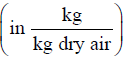is ___________.

(Important - Enter only the numerical value in the answer)

Detailed Solution for Mechanical Engineering - ME 2015 GATE Paper (Practice Test) - Question 20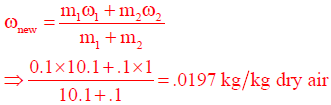*Answer can only contain numeric values
Mechanical Engineering - ME 2015 GATE Paper (Practice Test) - Question 21

Consider a steel (Young?s modulus E = 200 GPa) column hinged on both sides. Its height is 1.0m and cross-section is 10 mm × 20 mm. The lowest Euler critical buckling load (in N) is ______.

(Important - Enter only the numerical value in the answer)

Detailed Solution for Mechanical Engineering - ME 2015 GATE Paper (Practice Test) - Question 21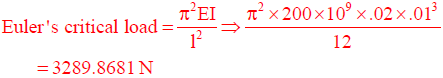*Answer can only contain numeric values
Mechanical Engineering - ME 2015 GATE Paper (Practice Test) - Question 22

Air enters a diesel engine with a density of 1.0 kg/m3. The compression ratio is 21. At steady state, the air intake is 30 × 10–3 kg/s and the net work output is 15 kW. The mean effective pressure (kPa) is _______.

(Important - Enter only the numerical value in the answer)

Detailed Solution for Mechanical Engineering - ME 2015 GATE Paper (Practice Test) - Question 22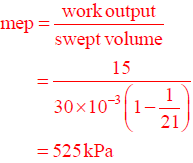Mechanical Engineering - ME 2015 GATE Paper (Practice Test) - Question 23

Match the following products with preferred manufacturing processes: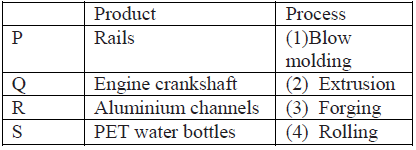*Answer can only contain numeric values
Mechanical Engineering - ME 2015 GATE Paper (Practice Test) - Question 24

Under certain cutting conditions, doubling the cutting speed reduces the tool life to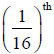of the original. Taylor?s tool life index(n) for this tool-workpiece combination will be _______

Detailed Solution for Mechanical Engineering - ME 2015 GATE Paper (Practice Test) - Question 24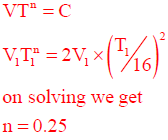Mechanical Engineering - ME 2015 GATE Paper (Practice Test) - Question 25

Consider a slider crank mechanism with nonzero masses and inertia. A constant torque τ is applied on the crank as shown in the figure. Which of the following plots best resembles variation of crank angle, θ versus time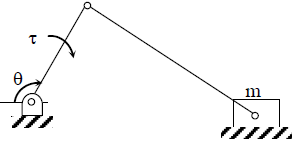Detailed Solution for Mechanical Engineering - ME 2015 GATE Paper (Practice Test) - Question 25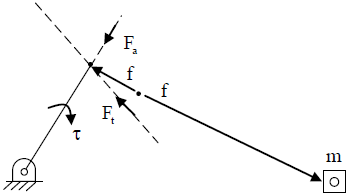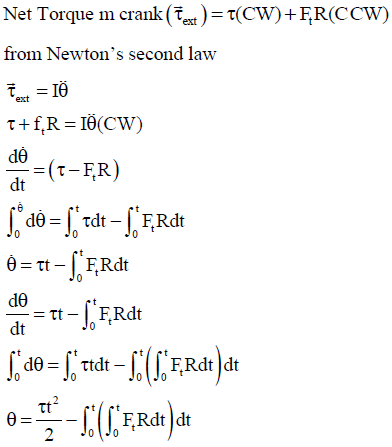Mechanical Engineering - ME 2015 GATE Paper (Practice Test) - Question 26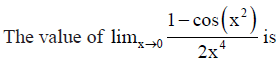Detailed Solution for Mechanical Engineering - ME 2015 GATE Paper (Practice Test) - Question 26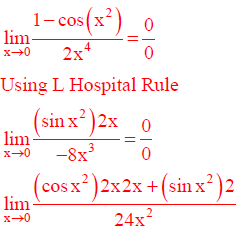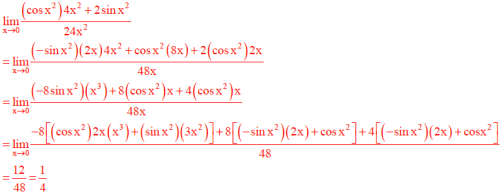*Answer can only contain numeric values
Mechanical Engineering - ME 2015 GATE Paper (Practice Test) - Question 27

Two identical trusses support a load of 100 N as shown in the figure. The length of each truss is 1.0 m, cross-sectional area is 200 mm2; Young?s modulus E = 200 GPa. The force in the truss AB (in N) is ______

(Important - Enter only the numerical value in the answer)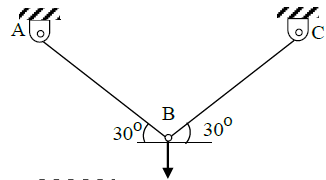Detailed Solution for Mechanical Engineering - ME 2015 GATE Paper (Practice Test) - Question 27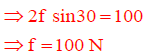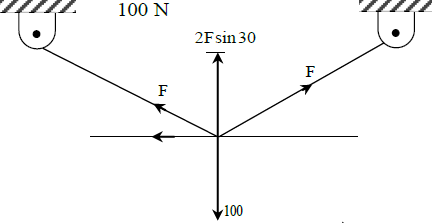Mechanical Engineering - ME 2015 GATE Paper (Practice Test) - Question 28

Among the four normal distributions with probability density functions as shown below, which one has the lowest variance?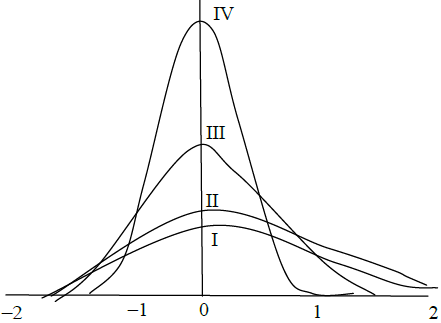Detailed Solution for Mechanical Engineering - ME 2015 GATE Paper (Practice Test) - Question 28

We have Probability distribution function of Normal Distribution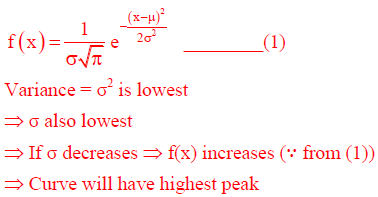Mechanical Engineering - ME 2015 GATE Paper (Practice Test) - Question 29

Given two complex numbers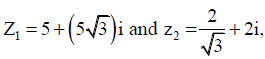the argument of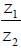in degrees is

Detailed Solution for Mechanical Engineering - ME 2015 GATE Paper (Practice Test) - Question 29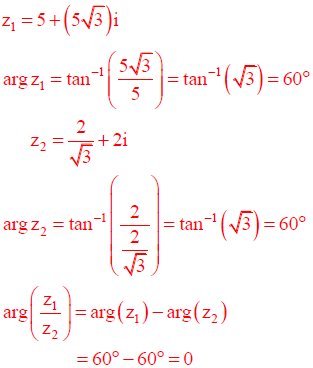Mechanical Engineering - ME 2015 GATE Paper (Practice Test) - Question 30

The Blasius equation related to boundary layer theory is a

*Answer can only contain numeric values
Mechanical Engineering - ME 2015 GATE Paper (Practice Test) - Question 31

A swimmer can swim 10 km in 2 hours when swimming along the flow of a river. While swimming against the flow, she takes 5 hours for the same distance. Her speed in still water (in km/h) is ________.

(Important - Enter only the numerical value in the answer)

Detailed Solution for Mechanical Engineering - ME 2015 GATE Paper (Practice Test) - Question 31

Let Swimmer = x

River = y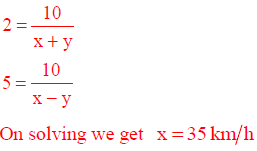Mechanical Engineering - ME 2015 GATE Paper (Practice Test) - Question 32

For flow of viscous fluid over a flat plate, if the fluid temperature is the same as the plate temperature, the thermal boundary layer is

Mechanical Engineering - ME 2015 GATE Paper (Practice Test) - Question 33

Which one of the following is the most conservative fatigue failure criterion?

Mechanical Engineering - ME 2015 GATE Paper (Practice Test) - Question 34

In a linear arc welding process, the heat input per unit length is inversely proportional to

Mechanical Engineering - ME 2015 GATE Paper (Practice Test) - Question 35

What is the deformation or dynamic factor if sum of errors on meshing teeth of steel pinion and gear is 32 x 10-3 mm?

Mechanical Engineering - ME 2015 GATE Paper (Practice Test) - Question 36

Q. No. 36 – 65 Carry One Mark Each

Q. A 10 mm diameter electrical conductor is covered by an insulation of 2 mm thickness. The conductivity of the insulation is 0.08 W/m-K and the convection coefficient at the insulation surface is 10 W/m2-K. Addition of further insulation of the same material will

Mechanical Engineering - ME 2015 GATE Paper (Practice Test) - Question 37

A machine element is subjected to the following bi-axial state of stress; σx = 80 MPa; σy = 20 MPa; τxy = 40MPa. If the shear strength of the material is 100 MPa, the factor of safety as per Tresca?s maximum shear stress theory is

Detailed Solution for Mechanical Engineering - ME 2015 GATE Paper (Practice Test) - Question 37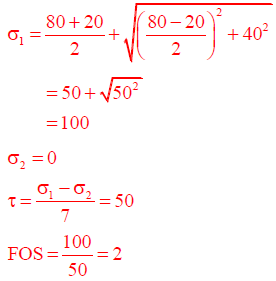Mechanical Engineering - ME 2015 GATE Paper (Practice Test) - Question 38

The probability of obtaining at least two “SIX” in throwing a fair dice 4 time is

Detailed Solution for Mechanical Engineering - ME 2015 GATE Paper (Practice Test) - Question 38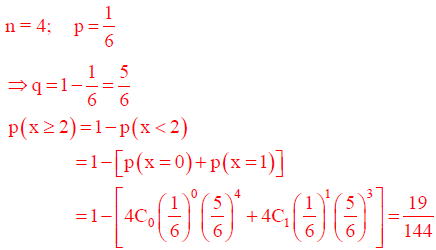*Answer can only contain numeric values
Mechanical Engineering - ME 2015 GATE Paper (Practice Test) - Question 39

A horizontal plate has been joined to a vertical post using four rivets arranged as shown in
figure. The magnitude of the load on the worst loaded rivet (in N) is ________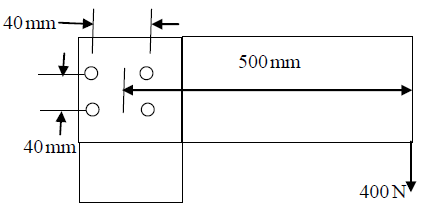(Important - Enter only the numerical value in the answer)

Detailed Solution for Mechanical Engineering - ME 2015 GATE Paper (Practice Test) - Question 39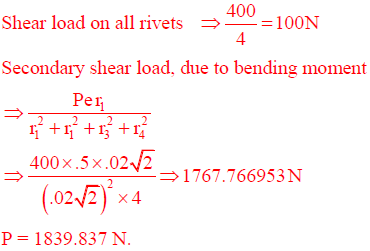*Answer can only contain numeric values
Mechanical Engineering - ME 2015 GATE Paper (Practice Test) - Question 40

Temperature of nitrogen in a vessel of volume 2 m3 is 288 K. A U-tube manometer connected to the vessel shows a reading of 70 cm of mercury (level higher in the end open to atmosphere). The universal gas constant is 8314 J/kmol-K, atmospheric pressure is 1.01325 bar, acceleration due to gravity is 9.81 m/s2 and density of mercury is 13600 kg/m3. The mass of nitrogen (in kg) in the vessel is _______.

(Important - Enter only the numerical value in the answer)

Detailed Solution for Mechanical Engineering - ME 2015 GATE Paper (Practice Test) - Question 40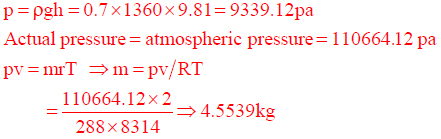Mechanical Engineering - ME 2015 GATE Paper (Practice Test) - Question 41

The solidification time of a casting is proportional to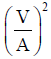, where V is the volume of the casting and A is the total casting surface area losing heat. Two cubes of same material and size are cast using sand casting process. The top face of one of the cubes is completely insulated. The ratio of the solidification time for the cube with top face insulated to that of the other cube is

Detailed Solution for Mechanical Engineering - ME 2015 GATE Paper (Practice Test) - Question 41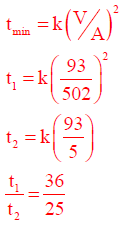Mechanical Engineering - ME 2015 GATE Paper (Practice Test) - Question 42

Match the following pairs: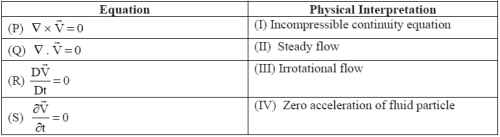*Answer can only contain numeric values
Mechanical Engineering - ME 2015 GATE Paper (Practice Test) - Question 43

Steam enters a well insulated turbine and expands isentropically throughout. At an intermediate pressure, 20 percent of the mass is extracted for process heating and the remaining steam expands isentropically to 9 kPa.

Inlet to turbine: P = 14 MPa, T = 560°C, h = 3486 kJ/kg, s = 6.6 kJ/(kg.K)

Intermediate stage: h = 27776 kJ/kg

Exit of turbine: P = 9 kPa, hf = 174 kJ/kg, hg = 2574 kJ/kg,

sf = 0.6 kJ/(kg.K), sg = 8.1 kJ/(kg.K)

If the flow rate of steam entering the turbine is 100 kg/s, then the work output (in MW) is _______.

(Important - Enter only the numerical value in the answer)

Detailed Solution for Mechanical Engineering - ME 2015 GATE Paper (Practice Test) - Question 43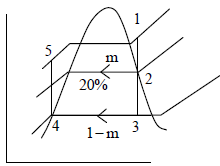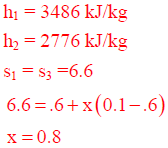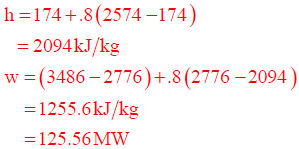*Answer can only contain numeric values
Mechanical Engineering - ME 2015 GATE Paper (Practice Test) - Question 44

Water (ρ = 1000 kg/m3) flows through a venturimeter with inlet diameter 80 mm and throat diameter 40 mm. The inlet and throat gauge pressures are measured to be 400 kPa and 130 kPa respectively. Assuming the venturimeter to be horizontal and neglecting friction, the inlet velocity (in m/s) is ________.

(Important - Enter only the numerical value in the answer)

Detailed Solution for Mechanical Engineering - ME 2015 GATE Paper (Practice Test) - Question 44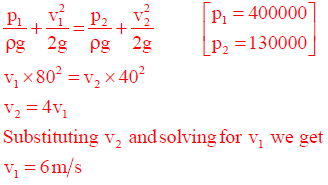*Answer can only contain numeric values
Mechanical Engineering - ME 2015 GATE Paper (Practice Test) - Question 45

For a canteen, the actual demand for disposable cups was 500 units in January and 600 units in February. The forecast for the month of January was 400 units. The forecast for the month of March considering smoothing coefficient as 0.75 is _______.

Detailed Solution for Mechanical Engineering - ME 2015 GATE Paper (Practice Test) - Question 45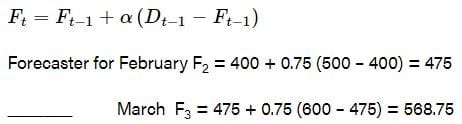*Answer can only contain numeric values
Mechanical Engineering - ME 2015 GATE Paper (Practice Test) - Question 46

Consider a spatial curve in three-dimensional space given in parametric form by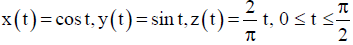The length of the curve is ______

(Important - Enter only the numerical value in the answer)

Detailed Solution for Mechanical Engineering - ME 2015 GATE Paper (Practice Test) - Question 46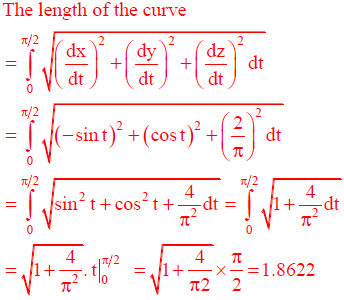Mechanical Engineering - ME 2015 GATE Paper (Practice Test) - Question 47

Considering massless rigid rod and small oscillations, the natural frequency (in rad/s) of vibration of the system shown in the figure is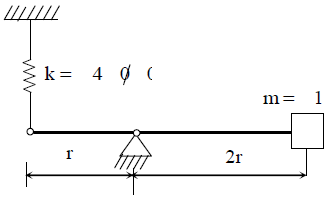Detailed Solution for Mechanical Engineering - ME 2015 GATE Paper (Practice Test) - Question 47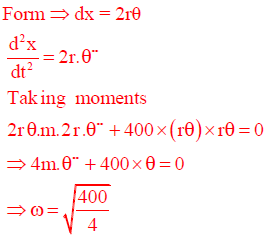Mechanical Engineering - ME 2015 GATE Paper (Practice Test) - Question 48

A triangular facet in a CAD model has vertices: P1(0, 0, 0); P2(1, 1, 0) and P3(1, 1, 1). The area of the facet is

Detailed Solution for Mechanical Engineering - ME 2015 GATE Paper (Practice Test) - Question 48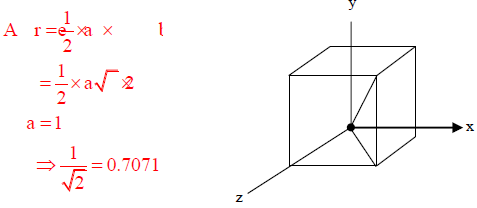*Answer can only contain numeric values
Mechanical Engineering - ME 2015 GATE Paper (Practice Test) - Question 49

In a slab rolling operation, the maximum thickness reduction (Δhmax) is given by Δhmax = μ2R, where R is the radius of the roll and μ is the coefficient of friction between the roll and the sheet. If μ = 0.1, the maximum angle subtended by the deformation zone at the centre of the roll (bite angle in degree) is ______.

(Important - Enter only the numerical value in the answer)

Detailed Solution for Mechanical Engineering - ME 2015 GATE Paper (Practice Test) - Question 49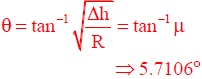Mechanical Engineering - ME 2015 GATE Paper (Practice Test) - Question 50

Find the solution of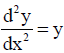which passes through the origin and the point (142,3/4)

Detailed Solution for Mechanical Engineering - ME 2015 GATE Paper (Practice Test) - Question 50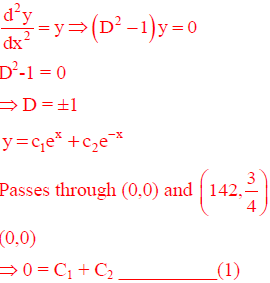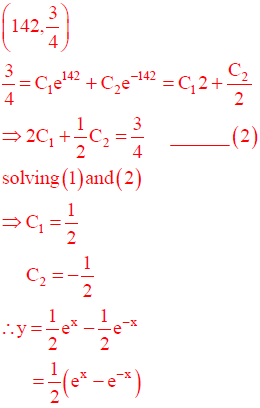Mechanical Engineering - ME 2015 GATE Paper (Practice Test) - Question 51

For the truss shown in figure, the magnitude of the force in member PR and the support reaction at R are respectively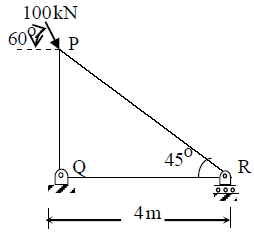Detailed Solution for Mechanical Engineering - ME 2015 GATE Paper (Practice Test) - Question 51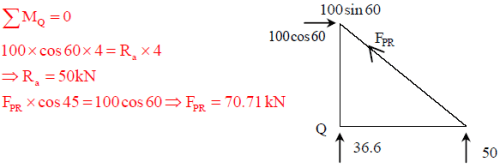*Answer can only contain numeric values
Mechanical Engineering - ME 2015 GATE Paper (Practice Test) - Question 52

A ball of mass 0.1 kg, initially at rest, is dropped from height of 1 m. Ball hits the ground and bounces off the ground. Upon impact with the ground, the velocity reduces by 20%. The height (in m) to which the ball will rise is ______

(Important - Enter only the numerical value in the answer)

Detailed Solution for Mechanical Engineering - ME 2015 GATE Paper (Practice Test) - Question 52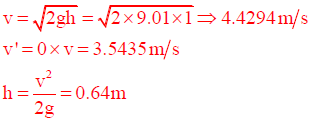*Answer can only contain numeric values
Mechanical Engineering - ME 2015 GATE Paper (Practice Test) - Question 53

A DC welding power source has a linear voltage-current (V-I) characteristic with open circuit voltage of 80 V and a short circuit current of 300 A. For maximum arc power, the current (in Amperes) should be set as _____.

(Important - Enter only the numerical value in the answer)

Detailed Solution for Mechanical Engineering - ME 2015 GATE Paper (Practice Test) - Question 53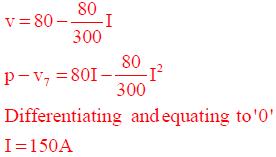Mechanical Engineering - ME 2015 GATE Paper (Practice Test) - Question 54

A well insulated rigid container of volume 1 m3 contains 1.0 kg of an ideal gas [Cp = 1000 J/(kg.K) and Cv = 800 J/(kg.K)] at a pressure of 105 Pa. A stirrer is rotated at constant rpm in the container for 1000 rotations and the applied torque is 100 N-m. The final temperature of the gas (in K) is _________.

(Important - Enter only the numerical value in the answer)

Detailed Solution for Mechanical Engineering - ME 2015 GATE Paper (Practice Test) - Question 54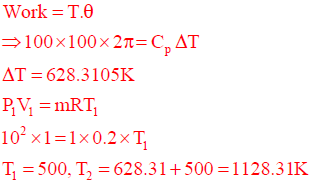Mechanical Engineering - ME 2015 GATE Paper (Practice Test) - Question 55

A pinion with radius r1, and inertia I1 is driving a gear with radius r2 and inertia I2. Torque τis applied on pinion. The following are free body diagrams of pinion and gear showing important forces (F1 and F2) of interaction. Which of the following relations hold true?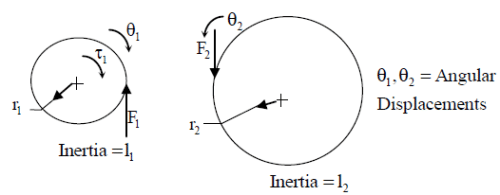Detailed Solution for Mechanical Engineering - ME 2015 GATE Paper (Practice Test) - Question 55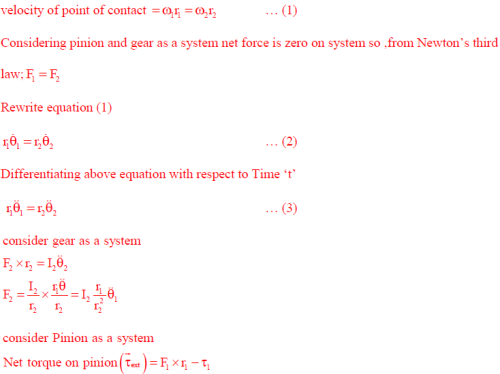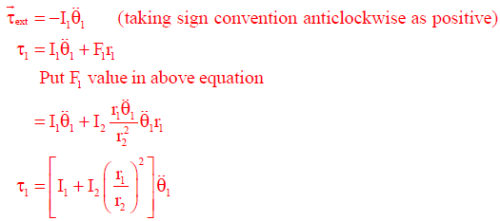*Answer can only contain numeric values
Mechanical Engineering - ME 2015 GATE Paper (Practice Test) - Question 56

A cantilever beam with flexural rigidity of 200 Nm2 is loaded as shown in the figure. The deflection (in mm) at the tip of the beam is _____.

(Important - Enter only the numerical value in the answer)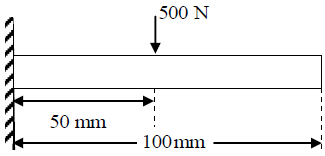Detailed Solution for Mechanical Engineering - ME 2015 GATE Paper (Practice Test) - Question 56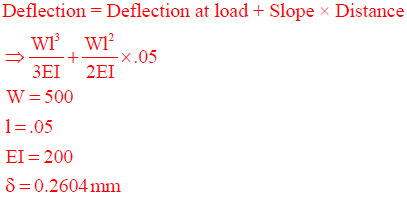Mechanical Engineering - ME 2015 GATE Paper (Practice Test) - Question 57

In the assembly shown below, the part dimensions are: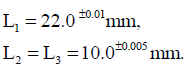Assuming the normal distribution of part dimensions, the dimension L4 in mm for assembly condition would be: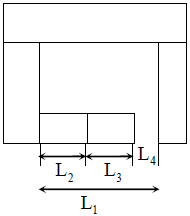Detailed Solution for Mechanical Engineering - ME 2015 GATE Paper (Practice Test) - Question 57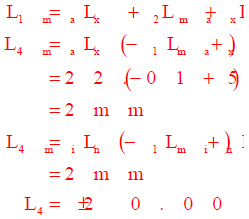Mechanical Engineering - ME 2015 GATE Paper (Practice Test) - Question 58

A mobile phone has a small motor with an eccentric mass used for vibrator mode. The location of the eccentric mass on motor with respect to center of gravity (CG) of the mobile and the rest of the dimensions of the mobile phone are shown. The mobile is kept on a flat horizontal surface.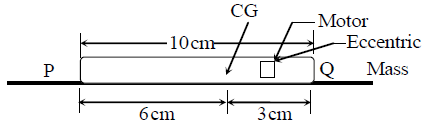Given in addition that the eccentric mass = 2 grams, eccentricty = 2.19 mm, mass of the mobile = 90 grams, g = 9.81 m/s2. Uniform speed of the motor in RPM for which the mobile will get just lifted off the ground at the end Q is approximately

Detailed Solution for Mechanical Engineering - ME 2015 GATE Paper (Practice Test) - Question 58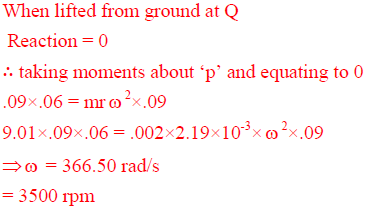*Answer can only contain numeric values
Mechanical Engineering - ME 2015 GATE Paper (Practice Test) - Question 59

A precision instrument package (m = 1 kg) needs to be mounted on a surface vibrating at 60 Hz. It is desired that only 5% of the base surface vibration amplitude be transmitted to the instrument. Assuming that the isolation is designed with its natural frequency significantly lesser than 60 Hz, so that the effect of damping may be ignored. The stiffness (in N/m) of the required mounting pad is ________.

(Important - Enter only the numerical value in the answer)

Detailed Solution for Mechanical Engineering - ME 2015 GATE Paper (Practice Test) - Question 59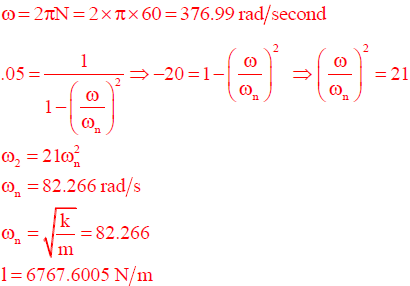Mechanical Engineering - ME 2015 GATE Paper (Practice Test) - Question 60

Following data refers to the activities of a project, where, node 1 refers to the start and node 5 refers to the end of the project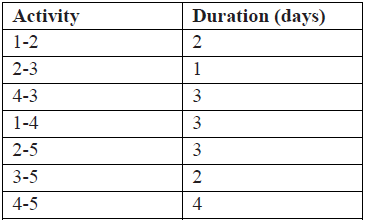The critical path (CP) in the network is

Detailed Solution for Mechanical Engineering - ME 2015 GATE Paper (Practice Test) - Question 60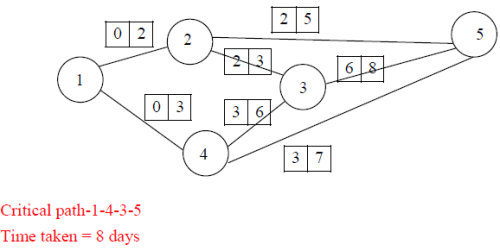Mechanical Engineering - ME 2015 GATE Paper (Practice Test) - Question 61

For flow through a pipe of radius R, the velocity and temperature distribution are as follows: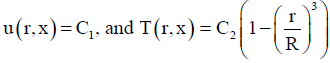,where C1 and C2 are constants
The bulk mean temperature is given by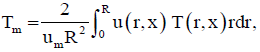with Um being the mean velocity of flow. The value of Tm is

Detailed Solution for Mechanical Engineering - ME 2015 GATE Paper (Practice Test) - Question 61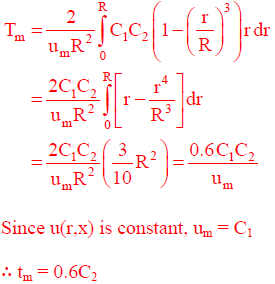*Answer can only contain numeric values
Mechanical Engineering - ME 2015 GATE Paper (Practice Test) - Question 62

Consider an ant crawling along the curve (x – 2)2 + y2 = 4, where x and y are in meters. The ant starts at the point (4, 0) and moves counter-clockwise with a speed of 1.57 meters per second. The time taken by the ant to reach the point (2, 2) is (in seconds) _______.

(Important - Enter only the numerical value in the answer)

Detailed Solution for Mechanical Engineering - ME 2015 GATE Paper (Practice Test) - Question 62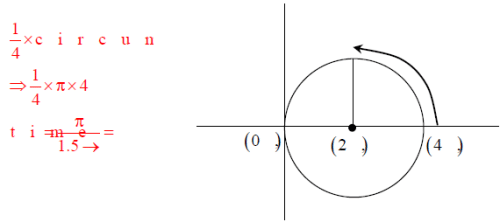*Answer can only contain numeric values
Mechanical Engineering - ME 2015 GATE Paper (Practice Test) - Question 63

Air (ρ = 1.2 kg/m3 and kinematic viscosity, v = 2 × 10–5 m2/s) with a velocity of 2 m/s flows over the top surface of a flat plate of length 2.5 m. If the average value of friction coefficient is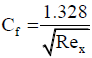, the total drag force (in N) per unit width of the plate is ________.

(Important - Enter only the numerical value in the answer)

Detailed Solution for Mechanical Engineering - ME 2015 GATE Paper (Practice Test) - Question 63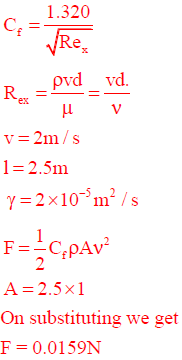*Answer can only contain numeric values
Mechanical Engineering - ME 2015 GATE Paper (Practice Test) - Question 64

The velocity field of an incompressible flow is given by

V = (a1x + a2y + a3z)i + (b1x + b2y + b3z)j + (c1x + c2y + c3z)k, where a1= 2 and c3 = – 4. The value of b2 is ________.

(Important - Enter only the numerical value in the answer)

Detailed Solution for Mechanical Engineering - ME 2015 GATE Paper (Practice Test) - Question 64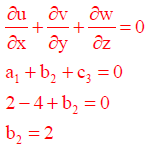*Answer can only contain numeric values
Mechanical Engineering - ME 2015 GATE Paper (Practice Test) - Question 65

An orthogonal cutting operation is being carried out in which uncut thickness is 0.010 mm, cutting speed is 130 m/min, rake angle is 15 and width of cut is 6 mm. It is observed that the chip thickness is 0.015 mm, the cutting force is 60 N and the thrust force is 25 N. The ratio of friction energy to total energy is __________

(correct to two decimal places)

Detailed Solution for Mechanical Engineering - ME 2015 GATE Paper (Practice Test) - Question 65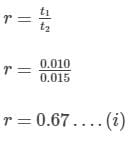F=FCsinα+FTcosα
F=60sin15+25cos15
F=39.67N….(ii)
Ratio of frictional enery to Total energy will be,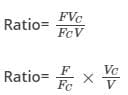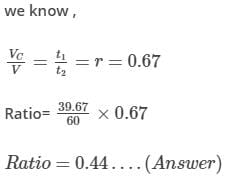## GATE Mechanical (ME) 2023 Mock Test Series

27 docs|243 tests
 Use Code STAYHOME200 and get INR 200 additional OFF Use Coupon Code
Information about Mechanical Engineering - ME 2015 GATE Paper (Practice Test) Page
In this test you can find the Exam questions for Mechanical Engineering - ME 2015 GATE Paper (Practice Test) solved & explained in the simplest way possible. Besides giving Questions and answers for Mechanical Engineering - ME 2015 GATE Paper (Practice Test), EduRev gives you an ample number of Online tests for practice

## GATE Mechanical (ME) 2023 Mock Test Series

27 docs|243 tests